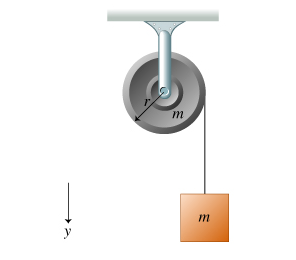# Problem: A string is wrapped around a uniform solid cylinder of radius r, as shown in the figure (Figure 1). The cylinder can rotate freely about its axis. The loose end of the string is attached to a block. The block and cylinder each have mass m. Note that the positive y direction is downward and counterclockwise torques are positive. Find the magnitude α of the angular acceleration of the cylinder as the block descends. Expressyour answer in terms of the cylinder's radius r and the magnitude of the acceleration due to gravity g.

###### FREE Expert Solution

Newton's second law:

$\overline{){\mathbf{\Sigma }}{\mathbf{F}}{\mathbf{=}}{\mathbf{m}}{\mathbf{a}}}$

Angular and linear accelerations are related by:

$\overline{){\mathbf{a}}{\mathbf{=}}{\mathbf{r}}{\mathbf{\alpha }}}$

Torque:

$\overline{){\mathbf{\tau }}{\mathbf{=}}{\mathbf{r}}{\mathbf{T}}}$

Moment of inertia for a cylinder:

$\overline{){\mathbf{I}}{\mathbf{=}}\frac{\mathbf{1}}{\mathbf{2}}{\mathbf{m}}{{\mathbf{r}}}^{{\mathbf{2}}}}$

Torque in terms of angular acceleration:

$\overline{){\mathbf{\tau }}{\mathbf{=}}{\mathbf{I}}{\mathbf{\alpha }}}$

Substituting for the moment of inertia and the expression of torque:

$\begin{array}{rcl}\mathbf{r}\mathbf{T}& \mathbf{=}& \mathbf{\left(}\frac{\mathbf{1}}{\mathbf{2}}{\mathbf{mr}}^{\mathbf{2}}\mathbf{\right)}\mathbf{\alpha }\\ \mathbf{T}& \mathbf{=}& \frac{\mathbf{1}}{\mathbf{2}}\mathbf{m}\mathbf{r}\mathbf{\alpha }\end{array}$

95% (284 ratings)###### Problem Details

A string is wrapped around a uniform solid cylinder of radius r, as shown in the figure (Figure 1). The cylinder can rotate freely about its axis. The loose end of the string is attached to a block. The block and cylinder each have mass m. Note that the positive y direction is downward and counterclockwise torques are positive.

Find the magnitude α of the angular acceleration of the cylinder as the block descends. Express
your answer in terms of the cylinder's radius r and the magnitude of the acceleration due to gravity g.